# Lecture Power Point Chemistry The Molecular Nature of

• Slides: 31Lecture Power. Point Chemistry The Molecular Nature of Matter and Change Seventh Edition Martin S. Silberberg and Patricia G. Amateis 5 -1 Copyright Mc. Graw-Hill Education. All rights reserved. No reproduction or distribution without the prior written consent of Mc. Graw-Hill Education.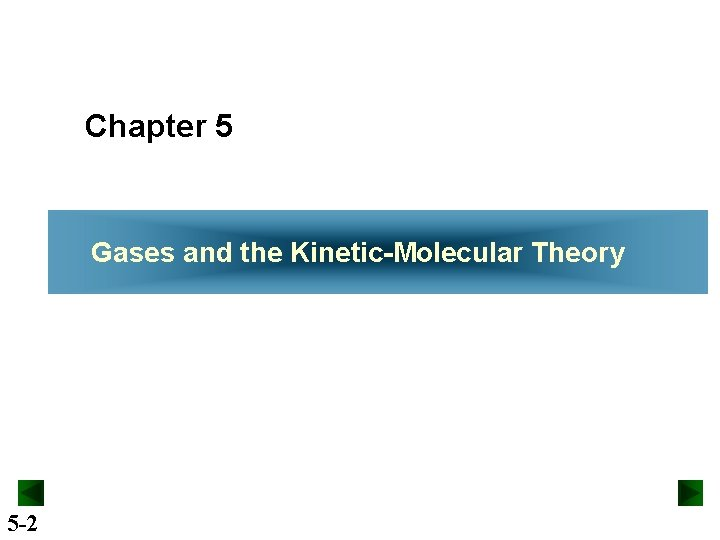Chapter 5 Gases and the Kinetic-Molecular Theory 5 -2Gases and the Kinetic Molecular Theory 5. 1 An Overview of the Physical States of Matter 5. 2 Gas Pressure and Its Measurement 5. 3 The Gas Laws and Their Experimental Foundations 5. 4 Rearrangements of the Ideal Gas Law 5. 5 The Kinetic-Molecular Theory: A Model for Gas Behavior 5. 6 Real Gases: Deviations from Ideal Behavior 5 -3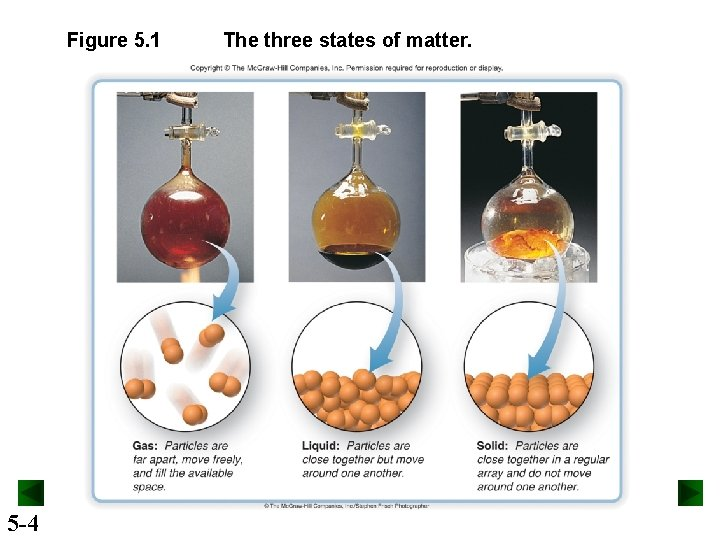Figure 5. 1 5 -4 The three states of matter.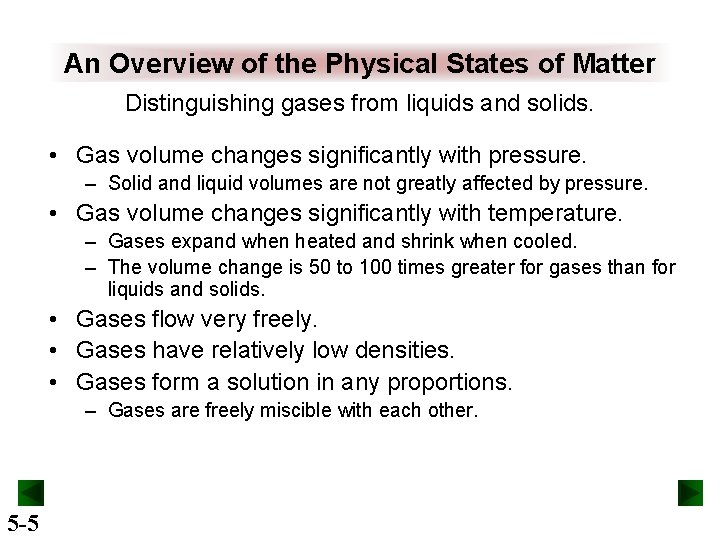An Overview of the Physical States of Matter Distinguishing gases from liquids and solids. • Gas volume changes significantly with pressure. – Solid and liquid volumes are not greatly affected by pressure. • Gas volume changes significantly with temperature. – Gases expand when heated and shrink when cooled. – The volume change is 50 to 100 times greater for gases than for liquids and solids. • Gases flow very freely. • Gases have relatively low densities. • Gases form a solution in any proportions. – Gases are freely miscible with each other. 5 -5Gas Pressure and its Measurement Pressure = force area Atmospheric pressure arises from the force exerted by atmospheric gases on the earth’s surface. Atmospheric pressure decreases with altitude. 5 -6Figure 5. 2 5 -7 Effect of atmospheric pressure on a familiar object.Figure 5. 3 A mercury barometer. https: //www. youtube. com/wat ch? v=Ek. Dhlz. A-lw. I 5 -8Figure 5. 4 A Closed-end manometer. The Hg levels are equal because both arms of the U tube are evacuated. 5 -9 A gas in the flask pushes the Hg level down in the left arm. The difference in levels, Dh, equals the gas pressure, Pgas.Figure 5. 4 B Open-end manometer. When Pgas is less than Patm, subtract Dh from Patm. Pgas < Patm Pgas = Patm - Dh 5 -10 When Pgas is greater than Patm, add Dh to Patm. Pgas > Patm Pgas = Patm + DhTable 5. 1 5 -11 Common Units of PressureSample Problem 5. 1 Converting Units of Pressure PROBLEM: A geochemist heats a limestone (Ca. CO 3) sample and collects the CO 2 released in an evacuated flask attached to a closed-end manometer. After the system comes to room temperature, Δh = 291. 4 mm Hg. Calculate the CO 2 pressure in torrs, atmospheres, and kilopascals. 5 -12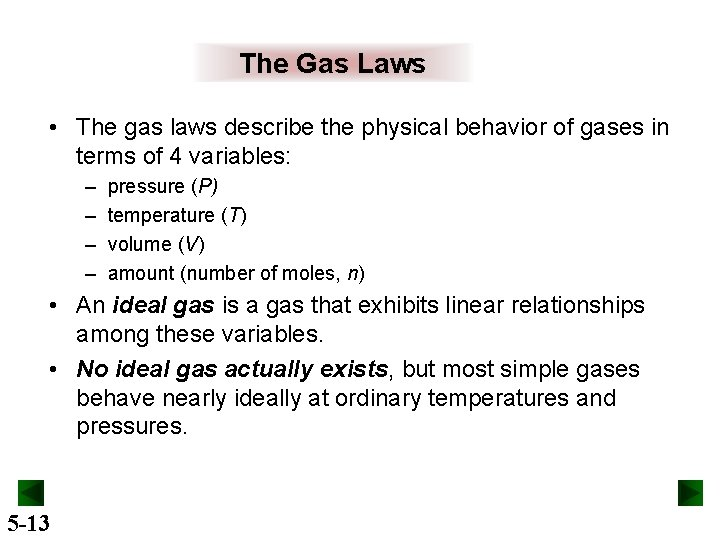The Gas Laws • The gas laws describe the physical behavior of gases in terms of 4 variables: – – pressure (P) temperature (T) volume (V) amount (number of moles, n) • An ideal gas is a gas that exhibits linear relationships among these variables. • No ideal gas actually exists, but most simple gases behave nearly ideally at ordinary temperatures and pressures. 5 -13Figure 5. 5 5 -14 Boyle’s law, the relationship between the volume and pressure of a gas.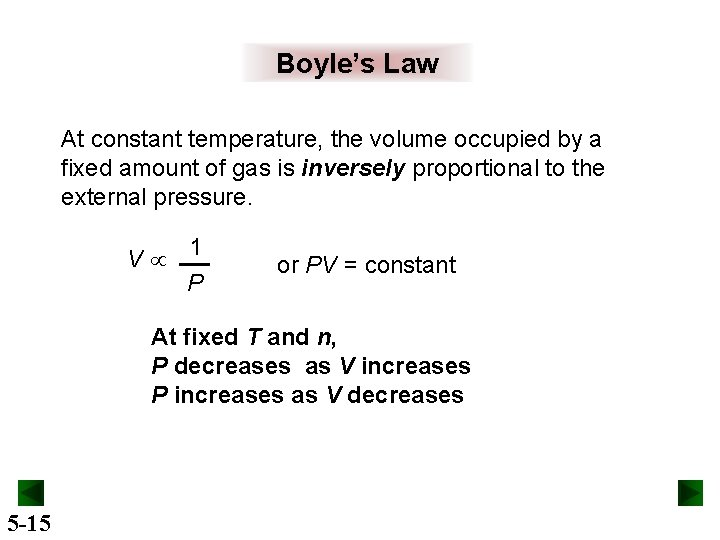Boyle’s Law At constant temperature, the volume occupied by a fixed amount of gas is inversely proportional to the external pressure. V 1 P or PV = constant At fixed T and n, P decreases as V increases P increases as V decreases 5 -15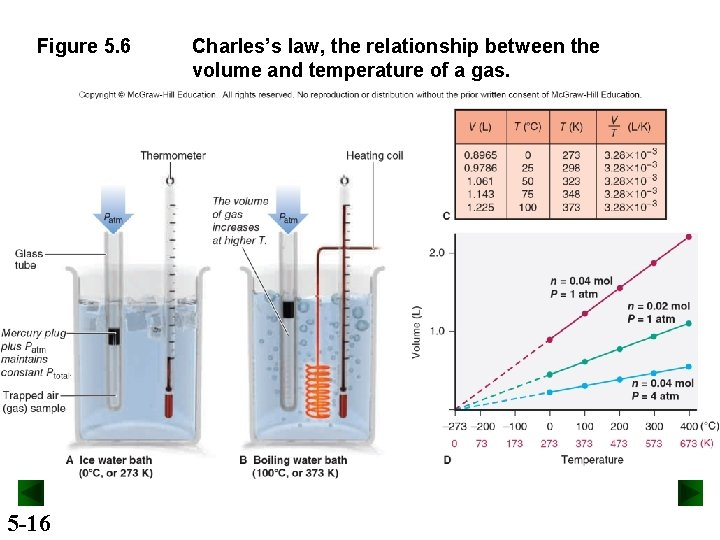Figure 5. 6 5 -16 Charles’s law, the relationship between the volume and temperature of a gas.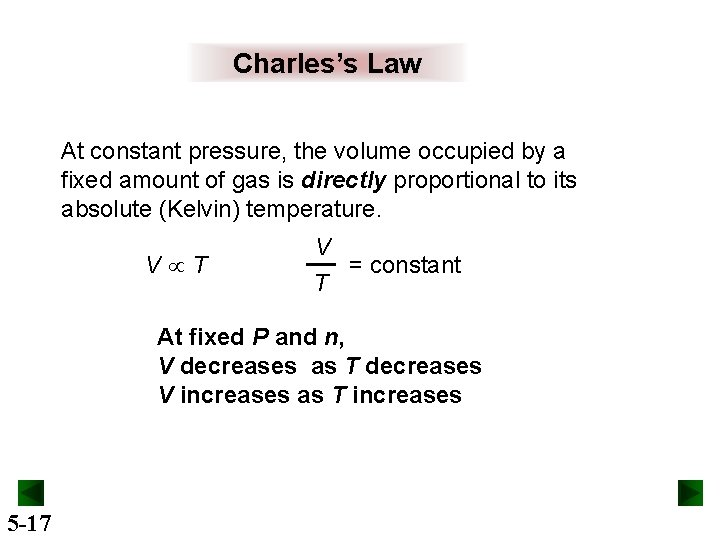Charles’s Law At constant pressure, the volume occupied by a fixed amount of gas is directly proportional to its absolute (Kelvin) temperature. V T V = constant T At fixed P and n, V decreases as T decreases V increases as T increases 5 -17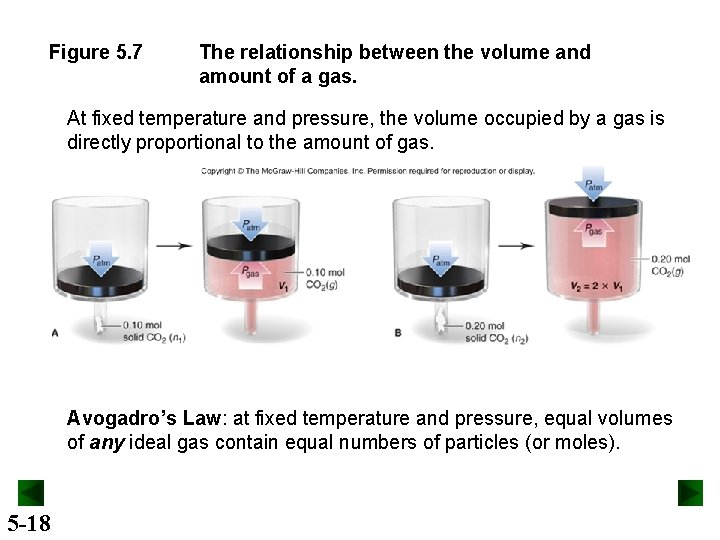Figure 5. 7 The relationship between the volume and amount of a gas. At fixed temperature and pressure, the volume occupied by a gas is directly proportional to the amount of gas. Avogadro’s Law: at fixed temperature and pressure, equal volumes of any ideal gas contain equal numbers of particles (or moles). 5 -18Figure 5. 8 5 -19 The process of breathing applies the gas laws.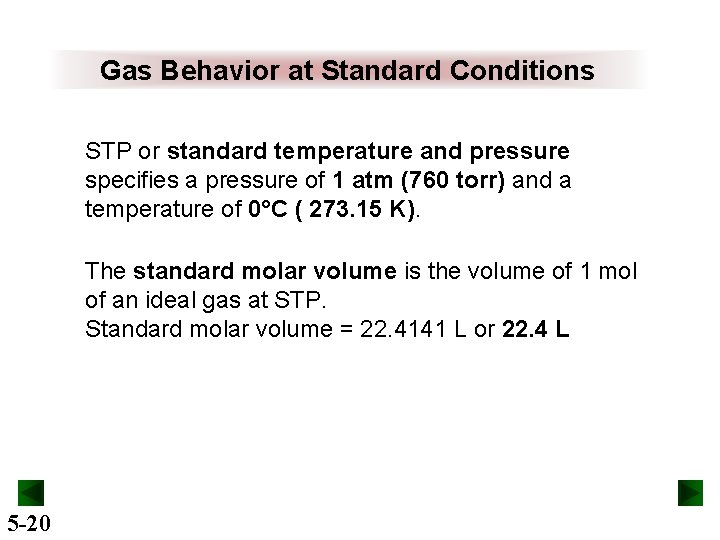Gas Behavior at Standard Conditions STP or standard temperature and pressure specifies a pressure of 1 atm (760 torr) and a temperature of 0°C ( 273. 15 K). The standard molar volume is the volume of 1 mol of an ideal gas at STP. Standard molar volume = 22. 4141 L or 22. 4 L 5 -20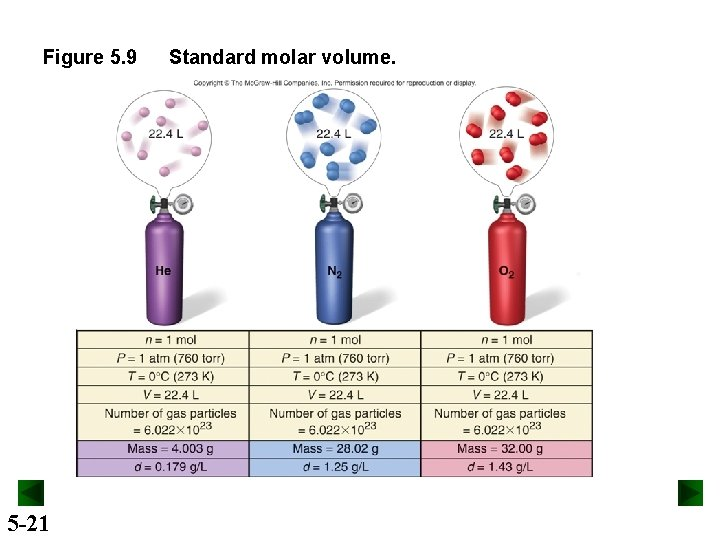Figure 5. 9 5 -21 Standard molar volume.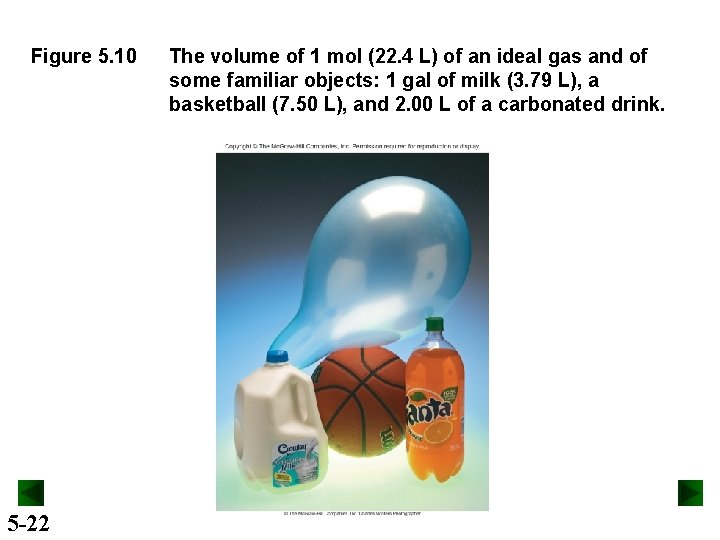Figure 5. 10 5 -22 The volume of 1 mol (22. 4 L) of an ideal gas and of some familiar objects: 1 gal of milk (3. 79 L), a basketball (7. 50 L), and 2. 00 L of a carbonated drink.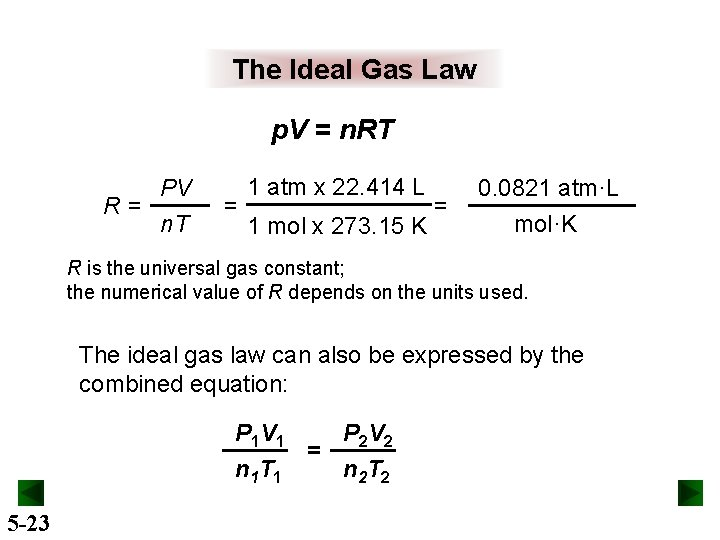The Ideal Gas Law p. V = n. RT PV R= n. T = 1 atm x 22. 414 L 1 mol x 273. 15 K = 0. 0821 atm·L mol·K R is the universal gas constant; the numerical value of R depends on the units used. The ideal gas law can also be expressed by the combined equation: P 1 V 1 P 2 V 2 = n 1 T 1 n 2 T 2 5 -23Figure 5. 11 5 -24 The individual gas laws as special cases of the ideal gas law.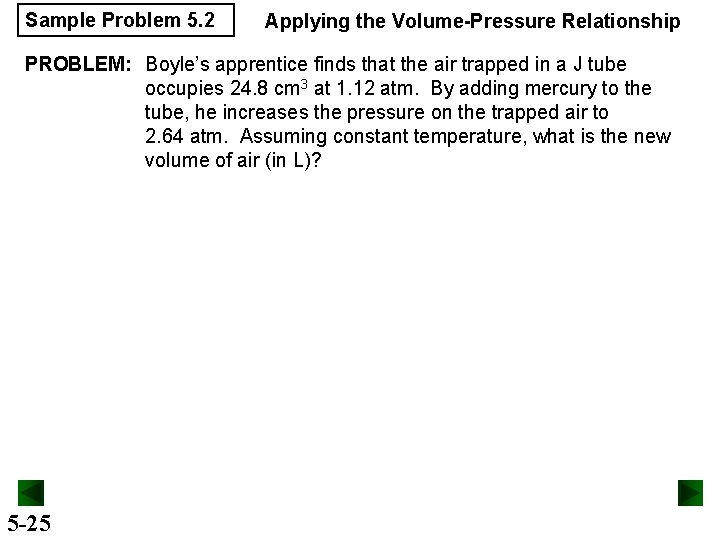Sample Problem 5. 2 Applying the Volume-Pressure Relationship PROBLEM: Boyle’s apprentice finds that the air trapped in a J tube occupies 24. 8 cm 3 at 1. 12 atm. By adding mercury to the tube, he increases the pressure on the trapped air to 2. 64 atm. Assuming constant temperature, what is the new volume of air (in L)? 5 -25Sample Problem 5. 3 Applying the Volume-Temperature and Pressure-Temperature Relationships PROBLEM: A balloon is filled with 1. 95 L of air at 25ºC and then placed in a car in the sun. What is the volume of the balloon when the temperature in the car reaches 90ºC? 5 -26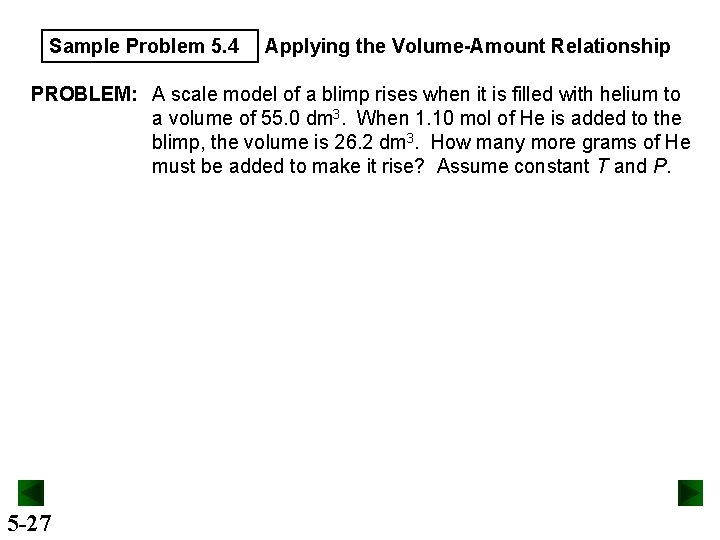Sample Problem 5. 4 Applying the Volume-Amount Relationship PROBLEM: A scale model of a blimp rises when it is filled with helium to a volume of 55. 0 dm 3. When 1. 10 mol of He is added to the blimp, the volume is 26. 2 dm 3. How many more grams of He must be added to make it rise? Assume constant T and P. 5 -27Sample Problem 5. 5 PROBLEM: 5 -28 Applying the Volume-Pressure-Temperature Relationship A helium-filled balloon has a volume of 15. 8 L at a pressure of 0. 980 atm and 22ºC. What is its volume on the top of Mt. Hood, Oregon’s highest mountain, where the atmospheric pressure is 532 mm. Hg and the temperature is 0ºC?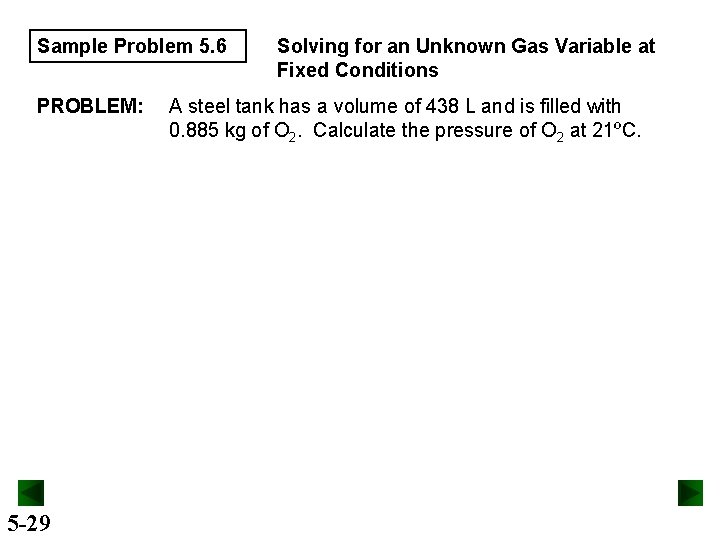Sample Problem 5. 6 PROBLEM: 5 -29 Solving for an Unknown Gas Variable at Fixed Conditions A steel tank has a volume of 438 L and is filled with 0. 885 kg of O 2. Calculate the pressure of O 2 at 21ºC.Sample Problem 5. 7 Using Gas Laws to Determine a Balanced Equation PROBLEM: The piston-cylinder is depicted before and after a gaseous reaction that is carried out at constant pressure. The temperature is 150 K before the reaction and 300 K after the reaction. (Assume the cylinder is insulated. ) Which of the following balanced equations describes the reaction? 5 -30 (1) A 2 (g) + B 2 (g) → 2 AB (g) (2) 2 AB (g) + B 2 (g) → 2 AB 2 (g) (3) A (g) + B 2 (g) → AB 2 (g) (4) 2 AB 2 (g) → A 2 (g) + 2 B 2 (g)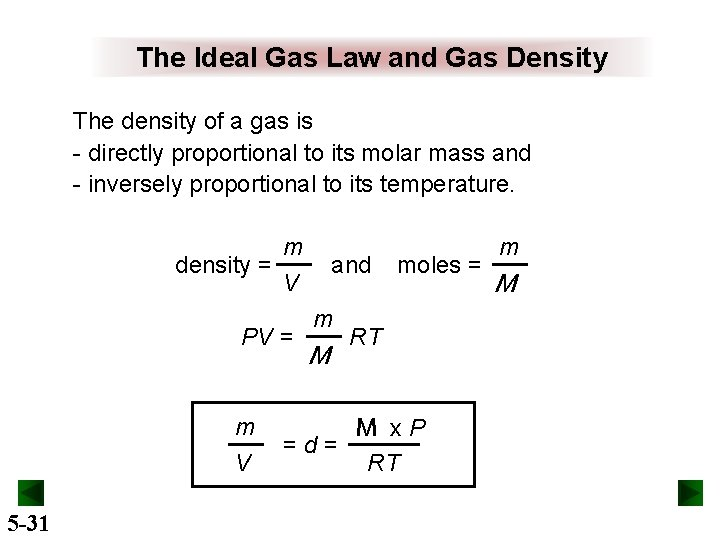The Ideal Gas Law and Gas Density The density of a gas is - directly proportional to its molar mass and - inversely proportional to its temperature. m density = V PV = m V 5 -31 and m M =d= moles = RT M x. P RT m M